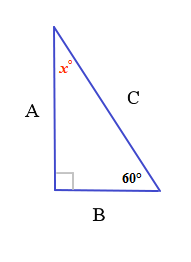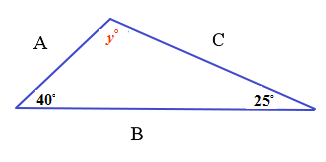Triangles: The Side-Angle Relationship

Triangles: The Side-Angle Relationship

What is the relationship between side B and angle x in the triangle below?Remember that the interior angles of a triangle add up to 180 degrees.

In this triangle, we then have 90+ 60+ x = 180=> 150+ x = 180=> x =180- 150=> x = 30So, the smallest angle is opposite the shortest side.

Let's look at another one:

What is the relationship between side B and angle y?40+ 25+ y = 180=> 65+ y = 180=> y= 115The largest angle is opposite the longest side.

These side-angle relationships are true for all types of triangles.